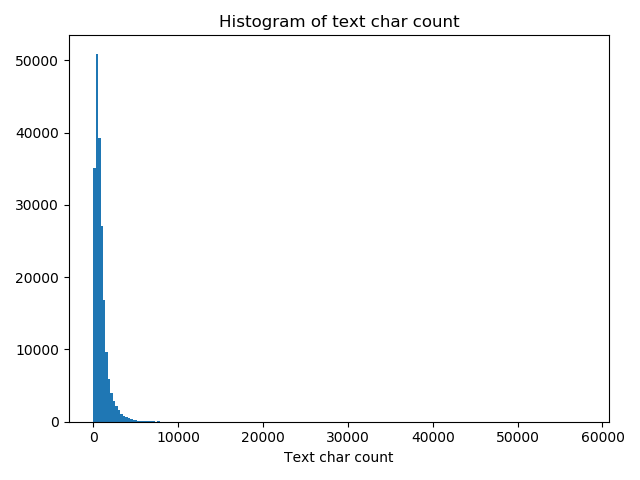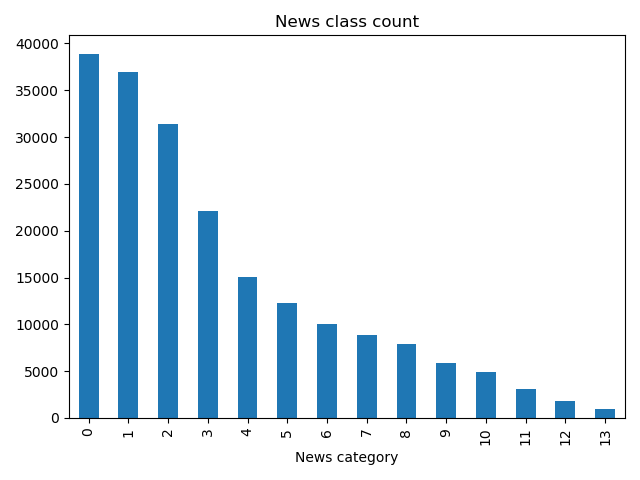# 零基础入门NLP - 天池新闻文本分类

### 数据读取

import pandas as pd


train_df.head()


ilabeltext
022967 6758 339 2021 1854 3731 4109 3792 4149 15…
1114464 486 6352 5619 2465 4802 1452 3137 5778 54…
237346 4068 5074 3747 5681 6093 1777 2226 7354 6…
327159 948 4866 2109 5520 2490 211 3956 5520 549…

### 数据分析

#### 句子长度分析

train_df['text_len'] = train_df['text'].apply(lambda x: len(x.split(' ')))
print(train_df['text_len'].describe())_ = plt.hist(train_df['text_len'], bins=200)
plt.xlabel('Text char count')
plt.title("Histogram of text char count")#### 新闻类别分析

train_df['label'].value_counts().plot(kind='bar')
plt.title('News class count')
plt.xlabel("News category")#### 字符分布统计

from collections import Counter
all_lines = ' '.join(list(train_df['text']))
word_count = Counter(all_lines.split(" "))
word_count = sorted(word_count.items(), key=lambda d:d, reverse = True)

print(len(word_count))
# 6869
print(word_count)
# ('3750', 7482224)
print(word_count[-1])
# ('3133', 1)


from collections import Counter
train_df['text_unique'] = train_df['text'].apply(lambda x: ' '.join(list(set(x.split(' ')))))
all_lines = ' '.join(list(train_df['text_unique']))
word_count = Counter(all_lines.split(" "))
word_count = sorted(word_count.items(), key=lambda d:int(d), reverse = True)

print(word_count)
# ('3750', 197997)
print(word_count)
# ('900', 197653)
print(word_count)
# ('648', 191975)


#### 结论

07-213407-2281
07-22114
07-2528
07-2739
07-2049
07-2170
07-3148
08-0436
10-16